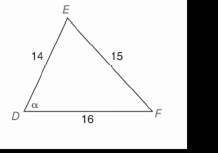Chapter 11.CR, Problem 10CRElementary Geometry For College St...

7th Edition
Alexander + 2 others
ISBN: 9781337614085

Solutions

Chapter
SectionElementary Geometry For College St...

7th Edition
Alexander + 2 others
ISBN: 9781337614085
Textbook Problem

In Review Exercises 9 to 12, use the Law of Sines or the Law of cosines to find the indicated length of side or angle measure. Angle measures should be found to the nearest degree; distances should be found to the nearest tenth of a unit.To determine

To find:

The indicated length of side or angle measure using the law of sines or the Law of Cosines.

Explanation

Procedure used:

In any triangle ABC such that

AB=c,

BC=a,

CA=b,

mA=α,

mB=β, and

mC=γ.

The Law of cosines is given by

a2=b2+c2-2bccosα

b2=c2+a2-2cacosβ

c2=a2+b2-2abcosγ

The Law of sines is given by

asinα=bsinβ=csinγ

Calculation:

Given,

From the above figure we have

a=15,

b=16, and

c=14

Also mEDF=α

By the law of cosine we have

a2=b2+c2-2bccosα

Substitute;a=15, b=16, and c=14. in the above formula

Still sussing out bartleby?

Check out a sample textbook solution.

See a sample solution

The Solution to Your Study Problems

Bartleby provides explanations to thousands of textbook problems written by our experts, many with advanced degrees!

Get Started

In Exercises 75-98, perform the indicated operations and/or simplify each expression. 86. (5x + 2)(3x 4)

Applied Calculus for the Managerial, Life, and Social Sciences: A Brief Approach

Fill in each blank: 6000floz=gal

Elementary Technical Mathematics

True or False: converges.

Study Guide for Stewart's Multivariable Calculus, 8th